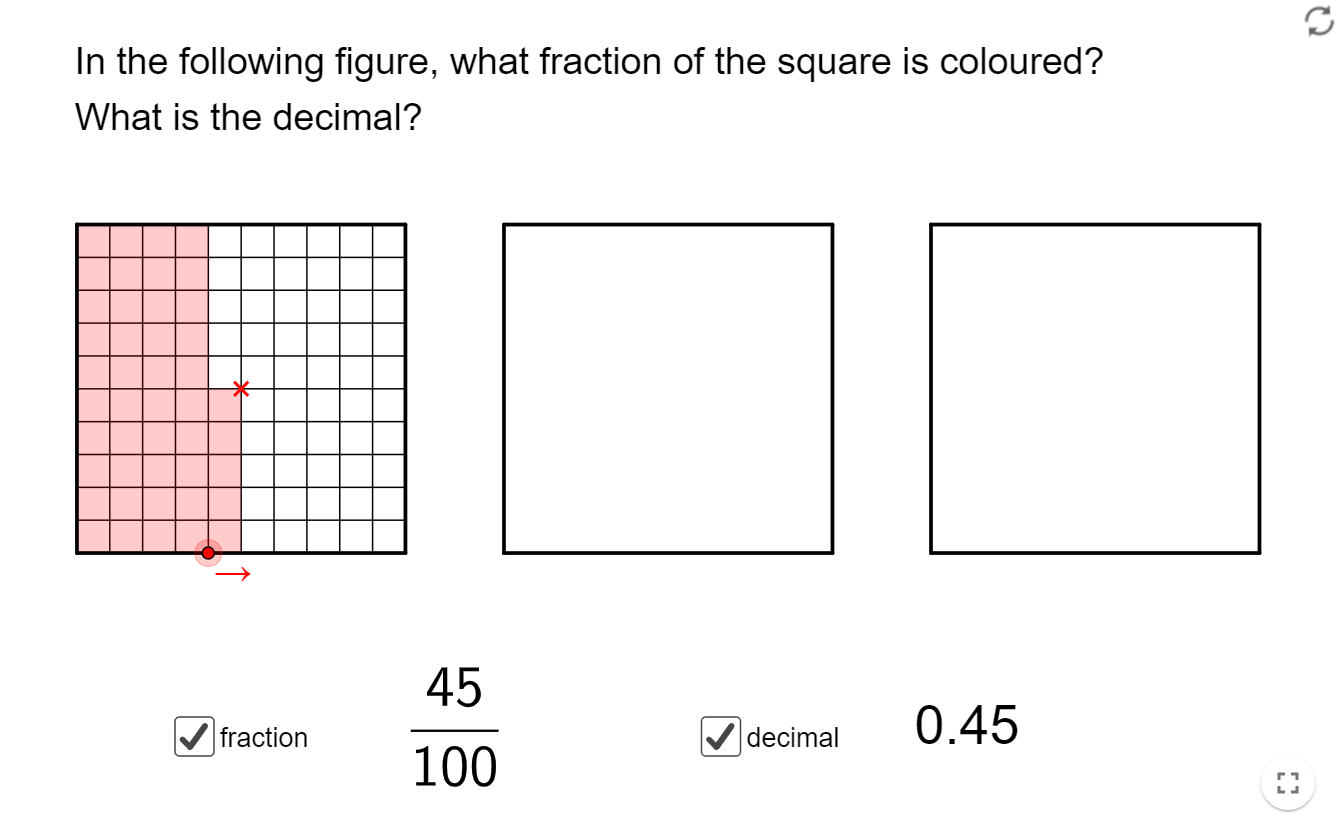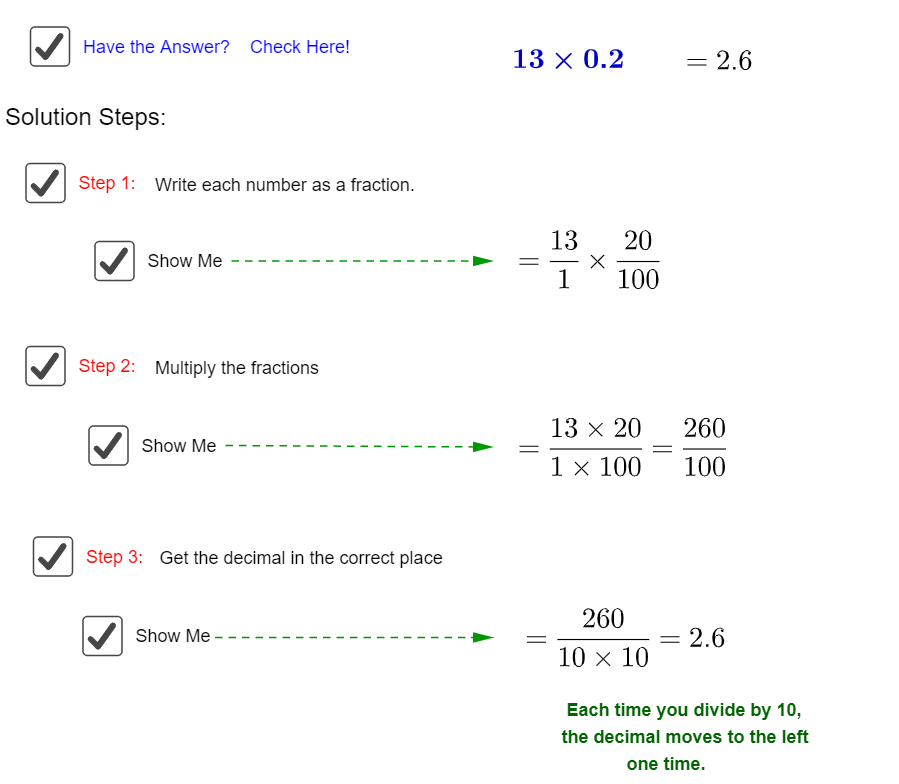# Multiplication of Decimals

Go back to  'Decimals'

## Introduction to Multiplication of Decimals

As we travel farther along in our mathematical journey, we see the basic operations being applied to almost all the new concepts that we pick up. The thing to keep in mind is that while they may seem different from the “basic” multiplication that was introduced to you earlier, but the difference is just at the surface. At the core of it, the governing principles still stay the same, and therein lies the beauty of math.

## The Big Idea: What is the Multiplication of Decimals?

Remember when you converted fractions to decimals and vice-versa? Well, if you are comfortable with multiplying fractions, then multiplying decimals becomes a walk in the park. It is essentially the same operation as it was for fractions!

Have a look at the image below and jog your memory with regards to the relationship between fractions and decimals:Essentially, you can treat multiplication of decimals as multiplication of fractions where the denominators are different multiples of 10!

Hence, when you multiply two decimals, you end up dividing the resulting number by the appropriate multiple of 10 based on how many decimal places the multiplicants had in total.

Here’s a simple example that would make it easier to visualise. Let us say you need to multiply 0.25 with 0.38 in which case these would be the steps:

\begin{align} & 0.25 \times 0.38\\ & = \frac{{25}}{{100}} \times \frac{{28}}{{100}}\\ & = \frac{{\left( {25 \times 38} \right)}}{{\left( {100 \times 100} \right)}}\\ & = \frac{{950}}{{10000}}\\ & = 0.095 \end{align}

Since the denominator had 4 zeroes we just had to move back the decimal point by four places. Let’s take a step back and review each step of the multiplication process again.### How to multiply decimals and whole numbers?

The fundamental principles of multiplication do not change. When multiplying a whole number with a decimal, simply treat the decimal number as a whole number and at the end divide the product with the appropriate multiple of 10 as dictated by the number of decimal places in the multiplicand. Here is a simple example to help elucidate the concept:

\begin{align}23 \times 1.943\end{align}

\begin{align} \;\;1.9\;4\;3\\ \underline {\;\;\;\; \times \;\,2\;3} \\ \;\;5.8\;2\;9\\ \underline {3\;8.8\;6\;0} \\ \underline {4\;4.6\;8\;9} \end{align}

Now the product above is what you would get if you multiply 1943 x 23. However, the decimal multiplicand was 1.943, in which case we divide 44689 by 1000 and the final product becomes: 44.689!

### How To Multiply Decimals greater than 1

The rules of the operation translate as it is even when the multiplicants are greater than 1. The same rule applies, convert to a fraction, in this case an improper fraction, multiply the numerator and denominator separately, then convert to decimal form. Here’s a simple example:

\begin{align} & 2.5 \times 3.12\\ & = \left( {\frac{{25}}{{10}}} \right) \times \left( {\frac{{312}}{{100}}} \right)\\ & = \frac{{\left( {25 \times 312} \right)}}{{\left( {10 \times 100} \right)}}\\ & = \frac{{7800}}{{1000}}\\ & = 7.800 \end{align}

As you can see, the total number of decimal digits were 3 in the multiplicants, and that remains conserved at the end with the product as well!

## How is it important?

### The Need for a Sanity Check

The problem many of us face while multiplying two decimal numbers which have a large number of numbers after the decimal point. This is true whether the two numbers to be multiplied are greater than 1 or less than 1.

When you are multiplying two numbers greater than 1, then do a quick multiplication of the whole number part of it to get a rough idea of the final answer. Let us say you need to multiply 2.08263 and 8.07261, then there is a possibility of the wrong answer being written if the decimal is wrongly moved back 9 places instead of 10 places, giving an answer 168.122597643, whereas the actual answer would be 16.8122597643. If you multiply 8 and 2 you would know that the final answer should be between 16 and 17.

More Important Topics
Numbers
Algebra
Geometry
Measurement
Money
Data
Trigonometry
Calculus
More Important Topics
Numbers
Algebra
Geometry
Measurement
Money
Data
Trigonometry
Calculus
Learn from the best math teachers and top your exams

• Live one on one classroom and doubt clearing
• Practice worksheets in and after class for conceptual clarity
• Personalized curriculum to keep up with school